# Error bar calculation (statistics)

## Homework Statement

For a project, I am trying to estimate the absorption curve of a certain plant species. Due to the variability within the species, I have taken the average of several measurements.

In the figure below, the blue curves represent 3 separate measurements, and the red curve is their average.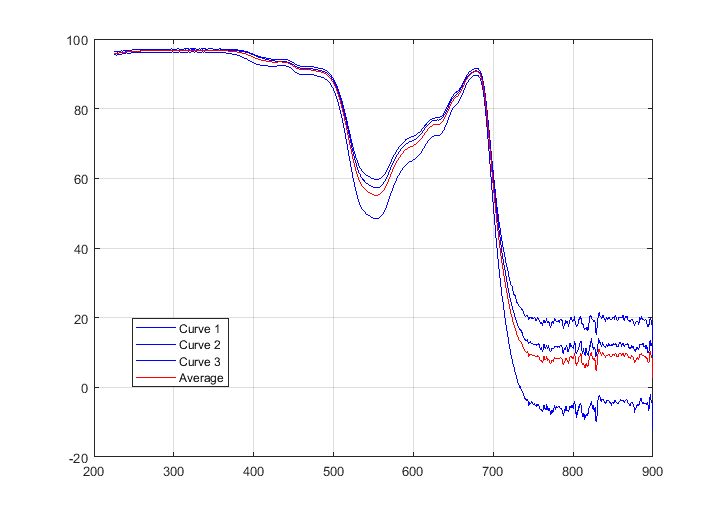I want to shade an area around the resulting red curve as a kind of error bound to represent the observed variability. What quantity do I need to consider here?

## The Attempt at a Solution

Should I simply shade the area from the lowest to the highest recorded value? Or is there some statistical parameter that I could use instead?In reality, I have taken much more measurements than the three curves shown above. I would like to know the most appropriate way to represent the magnitude of the error bars.

Any suggestions would be greatly appreciated.

#### Attachments

BvU
Homework Helper
Hi,

You want to pick up an introductory statistics textbook to introduce yourself to the subject.

Here you have done the averaging point by point using ##<x> = {1\over N}\displaystyle \sum_1^N x_i \ ## where ##<x>## indicates the average value.

The standard deviation estimate tells you where you expect 68% of the measurements to be: in the range ## [<x> - s_N, <x>+s_N]##

This ##s_N## ( aka ##\sigma_N##) divided by ##\sqrt N## tells you the estimated error in the average.

There is one snag: very close to 100% you can't expect symmetric Gaussian distributions; fortunately there your error bars are very small already.

PS are these absorption curves or transmission curves ?

•roam
Hi BvU,

Thanks a lot. These are absorptance curves — the fraction of light which is taken up by a given sample. One has to find both the transmittance ##\mathcal{T}## and reflectance ##\mathcal{R}## spectrophotometric curves. The absorptance ##\mathcal{A}## is then calculated from: ##\mathcal{A}=1-\mathcal{T}-\mathcal{R}##.

So, I have followed what you said. At each point, I calculated the standard deviation for the 3 values, then for the error I used ##\frac{1}{\sqrt{3}}\sigma_{N=3}##. This is what the result looks like: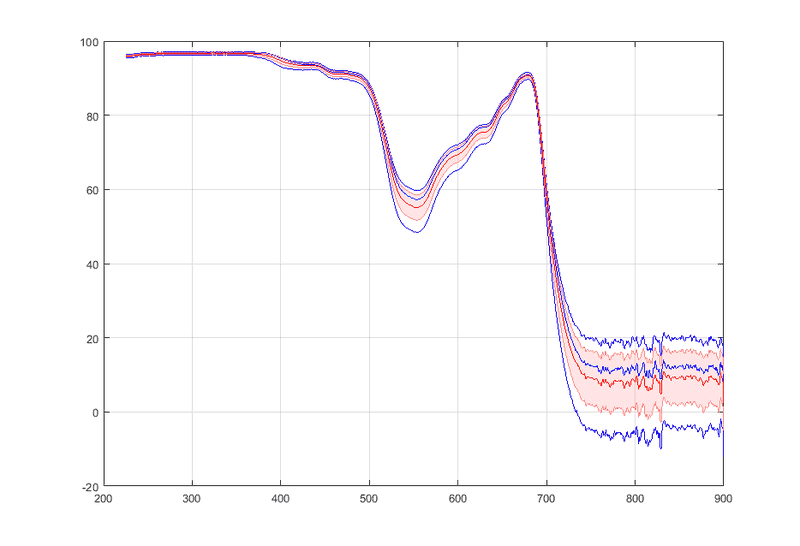The shaded red area is the values found from ##\frac{1}{\sqrt{3}}\sigma_{N=3}##, the red line is the mean, and the blue curves are the original data. Does this look about right?

There is one snag: very close to 100% you can't expect symmetric Gaussian distributions; fortunately there your error bars are very small already.

Could you explain this a bit more? Did you mean that the error bars would not be symmetric?Thanks a lot for the help. I don't have a background in statistics and found myself a bit swamped by all the info out there.

#### Attachments

BvU
Homework Helper
These are absorptance curves — the fraction of light which is taken up by a given sample
Yes, I looked at some of your recent threads out of sheer curiosity.

It does.

Actually I was referring to your
I have taken much more measurements than the three curves shown above
for ##N=3## there isn't much statistics.

And there are some remarks to be made:
The statistics expressions apply to independent observations. From the plots it looks like there is some correlation in the tail on the right, where you are somewhat in the noise ?

Did you mean that the error bars would not be symmetric?
Yes. A little. The expected distribution is no longer gaussian. Gaussians theoretically extend over all values, but your observations are limited to be ##\le## 100%.
But since you are several ##\sigma_m## away from 100% it's no big deal: the error in the size of the error bars will be greater than the possible asymmetry.

•roam
Ray Vickson
Homework Helper
Dearly Missed

## Homework Statement

For a project, I am trying to estimate the absorption curve of a certain plant species. Due to the variability within the species, I have taken the average of several measurements.

In the figure below, the blue curves represent 3 separate measurements, and the red curve is their average.

View attachment 229319

I want to shade an area around the resulting red curve as a kind of error bound to represent the observed variability. What quantity do I need to consider here?

## The Attempt at a Solution

Should I simply shade the area from the lowest to the highest recorded value? Or is there some statistical parameter that I could use instead?In reality, I have taken much more measurements than the three curves shown above. I would like to know the most appropriate way to represent the magnitude of the error bars.

Any suggestions would be greatly appreciated.

I would be very suspicious about applying standard statistical tests to these curves (to determine error bars, etc.). Here is why: it looks to me as though the three blue curves differ by some systematic factors, not just randomness. Where one curve has a (local) peak, so do the other two; where one decreases. so do the other two. One curve is consistently the highest throughout the whole range; one is consistently lowest, and the third lies between the other two---all the way from one end to the other.

That is not the type of behavior one would expect to see just from random errors. Systematic (non-random) differences would lead to what you see.

•roam
BvU
Homework Helper
Beg to differ a bit. There are systematic errors associated with the individual curves that average out.

I agree that there are systematic errors that are the same for every curve -- and those do not average out (the observations are simply not independent).

Depends on the details: for example,
is the same cuvet used with different samples -- systematic. New cuvet for every sample (probably) -- random errors.
is the same light source used (probably) -- systematic

You know more about the setup than we do, like: are T and R determined simultaneously ? With similar devices ? can't distinguish T and R spectra in the other thread picture but I am surprised they are so close together. How do the correlations sow up there ? In both ? equally divided ?

And I mentioned the visible correlation in the infrared tail that Ray noticed too - but is it very relevant for your results to have accurate error bars there ? (considering that in the far ultraviolet you have no measurement below 220 nm and considerable absorptance there)

•roam
Hi BvU,

You know more about the setup than we do, like: are T and R determined simultaneously ? With similar devices ? can't distinguish T and R spectra in the other thread picture but I am surprised they are so close together. How do the correlations sow up there ? In both ? equally divided ?

The device uses an integrating sphere to measure T and R sequentially. The exact same instrument was used throughout the experiments.

Regarding the correlation in the IR portion: the following is how the individual curves look like prior to subtraction and averaging. Each colour represents a given sample (the dashed lines being T, and the solid lines R).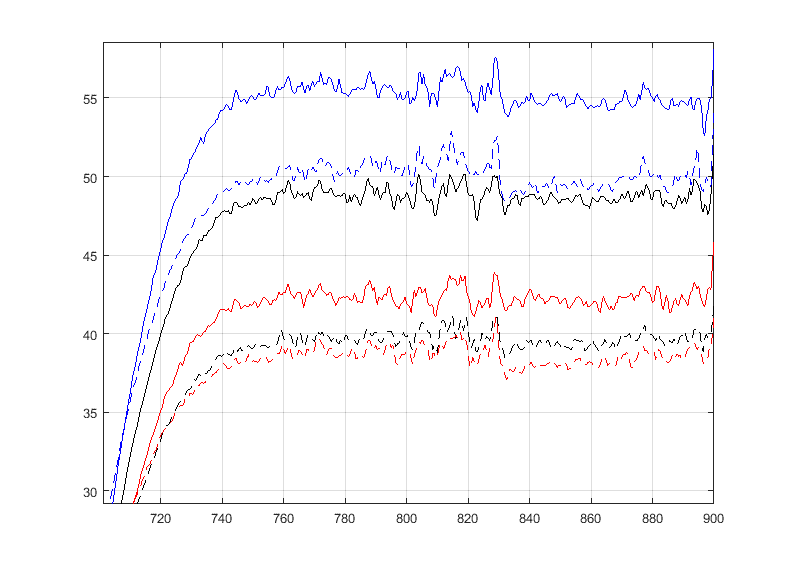They clearly look different when examined up close. The correlation is not strong. And we can't decisively say if the small amount of correlation is simply error, or a similar characteristic shared by the samples. I think these small bumps are inconsequential and can be flattened with smoothing — the point is that absorption is lowest in the NIR (how low depends on the sample).

The statistics expressions apply to independent observations.

Yes. Each time I measured a completely new leaf from a different plant (of the same species). Does that not qualify as independent observations? That's the best that we could do...

What would be a good minimum value for ##N##?

From the plots it looks like there is some correlation in the tail on the right, where you are somewhat in the noise ?

My spectrophotometer is really only intended for visible and UV, not IR. It starts to get noisy past ~750 nm, and with some numerical smoothing, you can get useful information up to 900 nm.

And I mentioned the visible correlation in the infrared tail that Ray noticed too - but is it very relevant for your results to have accurate error bars there ? (considering that in the far ultraviolet you have no measurement below 220 nm and considerable absorptance there)

I have two different species. I want to know whether it is possible to distinguish these two plants solely based on their chemical signature.

Of course, the absorption curves of all green plants have the same features (a superposition of the absorption of chlorophyll and water). On average however they seem to be a bit different. But when you add the error bars there is considerable overlap. This is partly why I need the error bars: to see how much overlap there is.

Is there some sort of criteria in statistics that would help you decide if the two sets of data are “distinguishable”?

For example, here is the absorbance for 4 different plant types (based on an average of 3 or 4 measurements):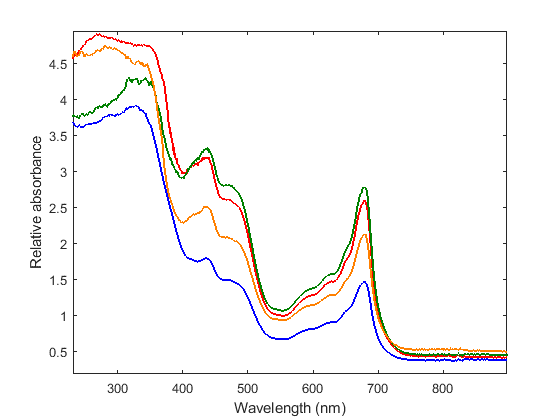#### Attachments

Ray Vickson
Homework Helper
Dearly Missed
Hi BvU,

The device uses an integrating sphere to measure T and R sequentially. The exact same instrument was used throughout the experiments.

Regarding the correlation in the IR portion: the following is how the individual curves look like prior to subtraction and averaging. Each colour represents a given sample (the dashed lines being T, and the solid lines R).

View attachment 229419

They clearly look different when examined up close. The correlation is not strong. And we can't decisively say if the small amount of correlation is simply error, or a similar characteristic shared by the samples. I think these small bumps are inconsequential and can be flattened with smoothing — the point is that absorption is lowest in the NIR (how low depends on the sample).

Yes. Each time I measured a completely new leaf from a different plant (of the same species). Does that not qualify as independent observations? That's the best that we could do...

What would be a good minimum value for ##N##?

My spectrophotometer is really only intended for visible and UV, not IR. It starts to get noisy past ~750 nm, and with some numerical smoothing, you can get useful information up to 900 nm.

I have two different species. I want to know whether it is possible to distinguish these two plants solely based on their chemical signature.

Of course, the absorption curves of all green plants have the same features (a superposition of the absorption of chlorophyll and water). On average however they seem to be a bit different. But when you add the error bars there is considerable overlap. This is partly why I need the error bars: to see how much overlap there is.

Is there some sort of criteria in statistics that would help you decide if the two sets of data are “distinguishable”?

For example, here is the absorbance for 4 different plant types (based on an average of 3 or 4 measurements):

View attachment 229420

Referring to your second graph (where you used 4 different plant types), it looks like you might have (significant?) systematic differences in results because the different plant types produce different curves (having the same general shapes but different levels). That is exactly the type of situation that statistical tools such as multivariate regression and/or "analysis of variance (ANOVA)" are designed to deal with. In ANOVA we seek to determine if differences in results for different "treatments" (plant types in your case) are real or just due to random chance events. You can also try to do that in a regression model. In a regression approach you might posit a model of the form
$$\text{Rel. abs.}(i,j) = a_i + f(\lambda_j) + \epsilon_{ij},$$
where ##a_1, a_2, a_3, a_4## are four different numbers corresponding to the four plant types ##i = 1,2,3,4## and the ##\lambda_j, j=1,2, \ldots, n## are the ##n## different wavelengths examined (the same for each plant type). The ##\epsilon_{ij}## are independent mean-zero random errors. Then, the statistical question becomes: after you have estimated the values of the ##a_i## from the data, are they truly 4 (or maybe only 3 or 2) different numbers, or are they 4 numbers that happen to look different because they are just a random sample of size four from some other type of error distribution?

If the ##a_i## are truly different, it would not make much sense to construct an error bar based on them. The way that error bars are interpreted in normal statistical practice is as some type of randomness range estimate. Thus, error bars about each of the four plots separately would be meaningful (assuming you have made enough measurements) because they would be telling you how reliable is the average curve for alfalfa (or oats, or wheat, or barley). Even if you fix attention on one type of crop and one wavelength, there are numerous other uncontrolled and uncontrollabe factors that make the results come out a bit different in each separate experimantal or observational run. That is what we try to treat as a random error, and drawing error bars around the alfalfa curve, or the barley curve, etc., makes good sense. What does not make much sense in some (but perhaps not all circumstances) is to construct some overall type of error bar based on the different crops, because that would essentially be assuming that farmers plant crops at random, tossing a die to decide whether to plant barley or wheat, etc. Of course, from a state-wide or country-wide view that MIGHT make sense, because while each farmer plants crops non-randomly, a large collection of farmers will appear to plant crops randomly. That is, if you were attempting to develop some type of "national" absorption curve, the four crop types would act as random factors that really do contribute to some overall "national" error bar.

Well, at least that is how I see it.

•roam and BvU
Hi Ray Vickson,

To give some context, my project is about automation distinguishing a specific plant from others in a pasture (e.g. using a hyperspectral camera).

For any species, there will be some degree of natural leaf-to-leaf variability. I am drawing error bars around the curve of each species to reflect this natural variation, rather than just measurement errors (errors of either systematic or accidental nature). By finding the average curves and their error bound, I want to know if it possible to positively determine the species of a given plant based on its spectrum.

So, if the ##a_i## are truly different (for a large enough sample size), can we use that to decide if they are distinguishable?

Also, I am assuming that ##f(\lambda)## is the underlying function. I doubt that it will be the same for all species (since the ingredients can differ slightly).

BvU
Homework Helper
to reflect this natural variation
Ah ! In that case you are interested in the standard deviation ##s_N## of the population, not the error in the average abdorptance ##\sigma_m \approx s_N/\scriptstyle \sqrt N\ ## !

And you are also interested in the accuracy of that estimate of this standard deviation, for which we have ##{\sigma(\sigma)\over \sigma} \approx {1\over\sqrt N}## (in other words: even with 100 observations you only have 10% accuracy in your error bar estimate)

Last edited:
•roam
Thank you for the clarification.

In my situation, measurement errors are small and not as conspicuous as the actual population variation. Using ##s_N## makes the error bounds larger. So if I understood correctly, we use the standard error ##s_N/\sqrt{N}## to only refer to the measurement errors, whereas ##s_N## itself accounts for the errors as well as the actual population variation. Is that correct?

P. S. In the last equation for ##\sigma(\sigma)/\sigma##, have you used the same definition as in the Wikipedia link? If so, I think it should be an equality sign rather than an approximation.

BvU
Homework Helper
If you want to distinguish between species, the hypothesis is: a (new) observation is compatible with the sample belonging to species X. In such a case the standard deviation in species X is relevant, not the (generally much smaller) error in the mean.

we use the standard error ##s_N/\sqrt{N}## to only refer to the measurement errors
No. To the calulated average.
The situation in your experiment is quite complicated: the determination of the absorptance spectrum of a species X at a wavelength ##\lambda## leads to a distribution that is spread out for at least two reasons:
samples from the species X have different absorptances, distributed with a standard deviation ##\sigma_x##
measurements have finite accuracy, the standard deviation is ##\sigma_o## (o for observation)​

so you measure a whole lot of representatives and get some distribution of absorptances. The distribution is close to gaussian so you apply the formulas to get an estimate of ##s_N##, the width of that distribution. If you are lucky, the two contribuants are independent and their ##\sigma##s can be added (in quadrature). You don't have a distinction between the two, unless you have a way to determine ##\sigma_o## separately.

Your measurements also yield the average absorptance ##a_X(\lambda)## The determination of the average has an error estimate of ##\sigma_m## which is hopefully small enough to ignore further on.

And you proceed with that ##a_X(\lambda)## and assume 68% of the measurements are within ##\pm s_N## from the average.

If so, I think it should be an equality sign rather than an approximation.
Yeah, well, it's statistics. I think that for ##N\uparrow \infty## the ##\approx## becomes a ##=## but I'm not quite certain...I should also concede here that I don't really know how to deal correctly (in a statistical sense) with hypothesis testing when one measurement is not a single number, but an entire spectrum.
Let alone the case when you are working with camera shots of pastures that may well ccontain a mixture of species ...

But you are definitely working on a subject that is relevant and very interesting !
##\mathstrut##

•roam
Is it possible to express ##s_N## as a linear sum of the different ##\sigma##s?

We could write ##\sigma_o## itself as a sum of noise and systematic errors. The systematic error can't be eliminated using mathematical procedures. But the accidental errors can be largely suppressed by global smoothing, for example by omitting high-frequency components in the Fourier spectrum which are purely caused by noise. But as you said ##\sigma_o<<\sigma_x##, so we can assume ##s_N \approx \sigma_x##.

Also, to be consistent I think it's better if we use ##s_N## everywhere for the sample standard deviation, so that ##s_N(s_N)/s_N \approx 1/\sqrt{N}##.

BvU
Homework Helper
Each time I measured a completely new leaf from a different plant (of the same species).
unless you have a way to determine ##\sigma_o## separately
It occurred to me that you might get an impression of that one by repeatedly measuring the same leaf from the same plant - preferably interleaved () with some other measurement.

But as you said ##\;\sigma_o<<\sigma_x##
I did ?
##\mathstrut##

•roam
It occurred to me that you might get an impression of that one by repeatedly measuring the same leaf from the same plant - preferably interleaved () with some other measurement.

Thanks a lot, I will try that! I think this might be more helpful for detecting the contribution of systematic errors. The errors due to noise (the jumpy parts) can simply be detected in Fourier space since noise has no tendency to converge.

I did ?

Sorry, you were referring to the error associated with the determination of the average. Absorption characteristics of leaves are not static physical constants and can vary quite a lot. If the measurement errors are greater than the actual natural variation... that means we have a very inaccurate measuring instrument.

Anyway, would I be on the right track collecting a large sample N? If the curves don't completely fall into one another's error bounds... that means we have a chance for assigning a plant into a species. (In your post #12, you said that the distribution would be Gaussian but that wouldn't be true if the sample size is very small)

For the small N, these are the profiles for two species: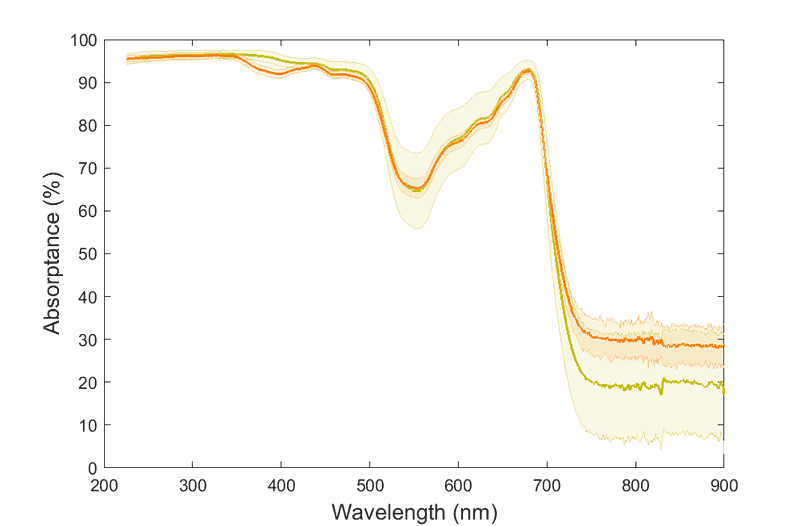The error bounds seem to continue to grow as N gets larger. How much overlap would mean that a new plant can no longer be assigned to a specific species?I'm wondering if there are some laws of statistics that come in operation here and can help to decide whether a distinction is possible.

#### Attachments

BvU
Homework Helper
Hi,

You really want to read up a little bit on hypothesis testing. As I said in #12, I have no good idea how to deal with spectra as opposed to scalars (numbers).

The error bounds seem to continue to grow as N gets larger
Should not be the case: if you increase a sample size, the estimate of the population standard deviation should become more accurate, but if it keeps growing there is something wrong.

I'd like to discuss the picture in #22, but "For the small N, these are the profiles for two species" doesn't contain much information. Suppose I see a green line with its ##s_{\scriptscriptstyle N}## band and an orange line with its ##s_{\scriptscriptstyle N}## band. Only range where they don't completely overlap is 380-400 nm and even there the difference is only three sigma. The remainder of the spectrum is useless for distinguishing between O and G.

Suppose (again) that somewhere in the range 380-400 nm the orange species O has 92 % ##\pm## 1 % absorptance and green G has 95 % ##\pm## 1 %.

If you now take one single measurement of an unknown species X and find 90 % ##\pm## 3% there are four hypotheses and each has a certain probability associated with it:
1. X is G
2. X is not G
3. X is O
4. X is not O
And with the values in this example given, none of them will stick out. 2 will be the biggest, 1 the smallest and 3 > 1.

•roam